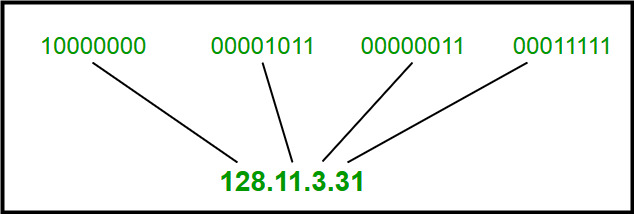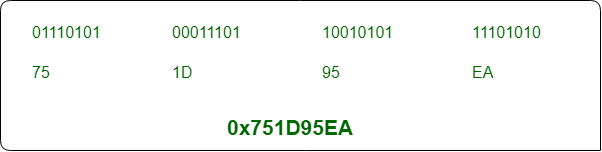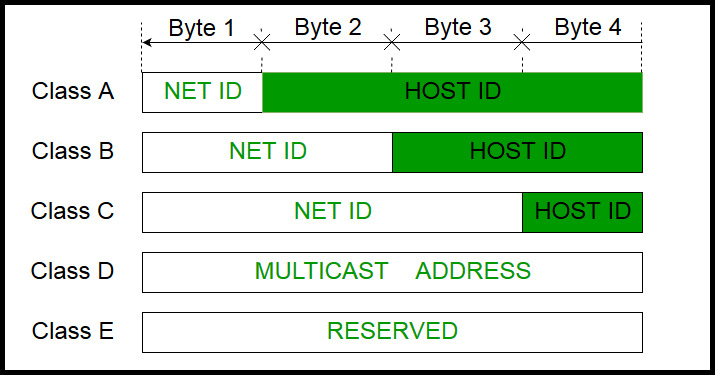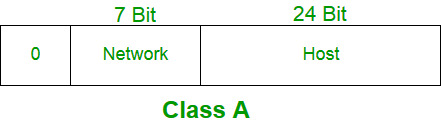Open in App
Not now

# What is the valid range of a Class A network address?

• Last Updated : 05 Jul, 2022

Let us know what an IP address is first, if you already know, skip this section.

IP (Internet Protocol):
It is one of the fundamental protocols inorder to have communications on the Internet. IP describes how the information is addressed, routed by networking devices. An IP address is a number identifying of a computer or another device on the Internet. A simple example could be mailing address.

```There are 2 types of IP
1. IPv4 : 32 bit long, Ex: 35.244.11.196
2. IPv6 : 128 bit long, it is so much longer than IPv4```

Example for IPv4 :

1. Dotted Decimal NotationIPv4: 32 bit long ( Dotted Decimal )IPv4: 32 bit long ( Hexadecimal )

• So, In IPv4 addressing, there are 5 classes to range IP Values : Class A, B, C, D and E.
• The order of bits in the first octet determine the classes of IP address.
• IPv4 address is divided into two parts:
1. Network ID
2. Host ID
• The class can determine the bits used for network ID and host ID.
• The number of total networks and hosts possible in that particular class can be calculated.
• Only class A, B and C are commonly used. classes D and E are reserved classes, D for multicast groups and E for future purposes.CLASS A :

• IP address belonging to class A are assigned to the networks that contain a large number of hosts. Here, class A can support 16 million hosts on 127 networks.
• Here, the network ID is 8 bits long and the host ID is 24 bits long.
• IP addresses belonging to class A ranges from 1.x.x.x – 126.x.x.x```Number Of Hosts and Networks:

Number of Hosts: (2^24)-2= 16,777,214 i.e 16 million hosts
Number of Networks: (2^7)-2=126
Note: Reducing 2 because, 0.0.0.0 and 127.a.b.c are different addresses```

>> The range of class A is 1.0.0.1 to 126.255.255.254

Other classes

Class B: 128.1.0.1 to 191.255.255.254
Class C: 192.0.1.1 to 223.255.254.254
Class D: 244.0.0.0 to 239.255.255.255
Class E: 240.0.0.0 to 254.255.255.254

My Personal Notes arrow_drop_up